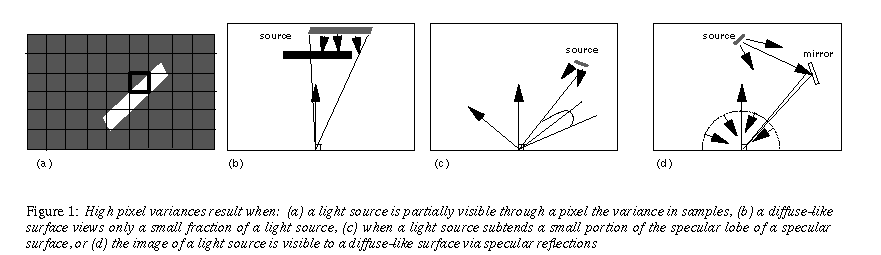## The Origin of Noisy Regions

A ``worst case'' estimate of the minimum number of trials Mt required for applying the test in Eq. 6 is given by Purgathofer . Scaling the values so that samples range from 0 to 1, the number of trials required is:

-For an image anti-aliasing problem with samples that ranged in value from 0 to 255, Purgathofer found that useful results were obtained when an interval of D = +/- 13 (i.e. d = 13/255=.05 in Eq. 8) was allowed with a confidence of 80%. These values of d and alpha give a minimum sampling of 32 trials/pixel.

The sampling rates required when computing radiance in ``real world'' floating point values and subsequently mapping to the device with a tone operator are much higher. While the form of tone operators varies, a typical radiance value on the order of 0.001 times the light source radiance is mapped in Eq. 3 to a value Np on the order of 100. An interval on the order of +/- 10 in the final display then requires an interval d equal to 0.0001 times the light source radiance. Scaling the problem so that the light source radiance is 1, a value of d = 0.0001 with a confidence of 80 %in Eq. 8 gives a minimum sampling rate of 16,094 trials per pixel!

The reorganization of the equation of transport given in Eq. 7 reduces the variance so that for most pixels in an image this worst case does not occur. However, there will be small regions in the image in which the ``worst case'' is encountered. These isolated regions will be noisy because they are undersampled. We summarize these high variance cases in Fig. 1.

Figure 1(a) illustrates the first type of high variance integration. Both light sources and non-light sources can be visible through some pixels. Sampling Eq. 2 for these pixels is essentially sampling a binomial distribution with several orders of magnitude in the two alternatives. Convergence is extremely slow in such cases.

-Figure 1(b) illustrates a second high variance case - the integration of direct illumination for a diffuse-like surface (first integral on the right of Eq. 7). For a point which has a full view of the light source, a small number of trials are needed to estimate the cosine and distance terms in the integral. However, when the view of a light source is partially obscured a large number of trials may be required to estimate the visible area. The smaller the fraction of the source that is visible, the larger the number of trials needed. Since a light source has a high radiance, just one ``hit'' will result in a large sample standard deviation.

Figure 1(c) illustrates the integration of reflected light for a specular-like surface, the third integral on the right of Eq. 7. The BRDF is concentrated on a small lobe near the specular direction. A high variance in samples for this case occurs when a small portion of this lobe is subtended by a light source.

The fourth type of integration with high deviations is the case of ``caustic paths'', shown in Fig. 1(d). The integral diagrammed in Figure 1(d) is the second term on the right of Eq. 7. A ``caustic'' appears when a small portion of the incident hemisphere is subtended by the image of a light source in a specular-like reflection. When a sample ray hits this small image, a large deviation in the sample is introduced.

In any of the four cases, high deviations are expected in some region of the scene, not at one point. Light source edges, penumbrae, fuzzy specular reflections and caustics spread through regions. For Q pixels in one of these regions, only a small number of pixels q will have obtained samples hitting the high radiance portion of the domain of integration. The q pixels will appear to be adding noise to the region of Q-q pixels that appear to be accurate. Actually, all Q pixels are equally valid. Rather than throwing out the q ``noise'' pixels, the true value that should have been calculated for the whole region should be an average of all Q values.